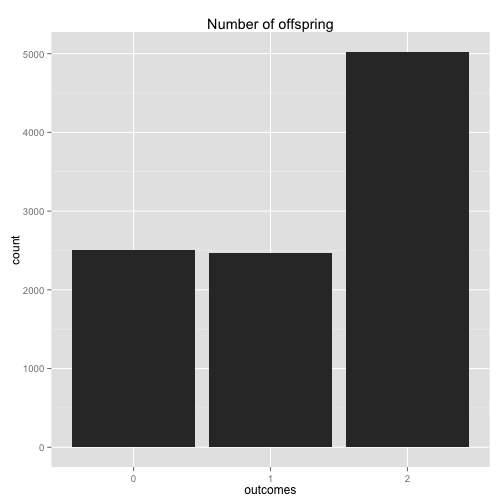# "Bobo the amoeba"

## Problem statement

Bobo the amoeba has a 25%, 25%, and 50% chance of producing 0, 1, or 2 offspring, respectively. Each of Bobo’s descendants also have the same probabilities. What is the probability that Bobo’s lineage dies out?

## Analytical solution

Let $$X$$ be a random variable that describes the event "the population eventually dies out". We are asked to find $$P(X)$$. For $$i$$ in $${0,1,2}$$ let $$A_i$$ be a r.v. describing the event that Bobo turns into $$i$$ amoebas. It follows that

1. $$P(X|A_0) = 1$$; Bobo dies without an offspring
2. $$P(X|A_1) = P(X)$$; Bobo has one offspring, which will retain its reproduction probabilities
3. $$P(A|X_2) = P(X)^2$$; Bobo has two offspring. Both will retain its reproduction probabilities

By the law of total probability we can now write $$P(X)$$ as

$$P(X) = \frac{1}{4} P(X|A_0) + \frac{1}{4} P(X|A_1) + \frac{1}{2} P(X|A_2)$$

by doing simple algebra we reduce the equation to $$0 = \frac{1}{4} + P(X) (-\frac{3}{4} + \frac{1}{2} P(X))$$

which has roots $$P(X) = \frac{1}{2}$$ and $$P(X) = 1$$.

Which one should we pick? Intuitively we can expect P(X) to be < 1, but let's validate this by means of simulation.

## Simulation

We can simulate a few iterations of Bobo's reproductive process and count how many times its lineage died out. If we do this for a large enough number of iterations, we should get an asymptotic value good enough to discriminate between the two roots. It's also a good excuse to brush up some R.

library('ggplot2')


We can encode the probabilities $$P(A_i)$$ as

p <- c(0.25, 0.25, 0.50)


To generate an offspring wrt the given proabilities p we use a function that simulates a multinomial distribution.

offspring <- function(v) {
r <- runif(n=1)
inP <- -1
for (k in 1:length(p)) {
inP <- k
if ((k == 1) & (r < v[k])) {
inP <- k-1
break
}
else if ( (k>1) & (r < sum(v[1:k])) ) {
inP <- k-1
break
}
}
return(inP)
}


We can refactor this code in a more performant, vectorized, form as:

offspring <- function(v) {
match(1, rmultinom(1, 1, p))-1
}


To convince ourselves that the multinomial simulation works, let's plot the frequency of each offspring over a few thousand iterations.

t <- replicate(10000, offspring(p))

df <- as.data.frame(t)
colnames(df) <- c("outcomes")
ggplot(data=df, aes(x=as.factor(outcomes))) +
geom_histogram() +
xlab("outcomes") +
ggtitle("Number of offspring")### Simulation runs

max_iterations <- 1000
max_generations <- 100


Finally let's simulate Bobo offspring over time. How many runs resulted in the offspring dying off within {r} max_generations out of {r} max_iterations?

is_extinct <-function(generations) {
next_offs <- 0 # offspring of the next generation

while (offs <= generations) { # loop through generations
if (offs == 0) { # lineage died out
break
}
next_offs <- 0 # offspring of the current generation
for (j in 1:offs) {
next_offs <- next_offs + offspring(p)
}
offs <- next_offs
}
return(as.integer(offs < generations)) # TRUE if the lineage dies out
}

# count the number of times Bobo's lineage died off. Do it for 10 runs of
# max_iterations each
res <- replicate(10,
sum(replicate(max_iterations, is_extinct(max_generations)))
/ max_iterations)


Results suggest that Bobo's lineage will go extinct with $$P(X) = \frac{1}{2}$$.

summary(res)

##    Min. 1st Qu.  Median    Mean 3rd Qu.    Max.
##  0.4820  0.4980  0.5015  0.5051  0.5130  0.5240


## Analytical solution, reprise

More formally, this problem can be modelled as a branching process; that is, we can look at it as a Markov chain where the state $$A_n$$ represents the number of amoebas at generation (or time) $$n$$, and transition probabilities can be written in the from $$P(A_{n+1} = i |A_n =k) = P(W_1 + W_2 + ... + W_k = i)$$, where $$W_1, ..., W_k$$ are independent random variables each representing the offspring distribution. This leads to a recursive representation of the problem, where each $$W_k$$ models a branching process. We observe that if $$A_n$$ reaches 0, it says there (0 is an absorbing state). It is known (theorem) that when an individual has less than one offspring on average, denoted as $$\mu \leq 1$$, its lineage will die out with $$P("extinction") = 1$$. When $$\mu \gt 1$$ there are two solutions for the recursive sequence $$P(X) = \frac{1}{4} P(X|A_0) + \frac{1}{4} P(X|A_1) + \frac{1}{2} P(X|A_2)$$ and the extinction probability is the smallest of the two. Analysis and proofs can be found at this link

In our case the offspring expectation is $$\mu = 1.25$$; it follows that Bobo's lineage will go extinct with probability $$P(X) = \frac{1}{2}$$.